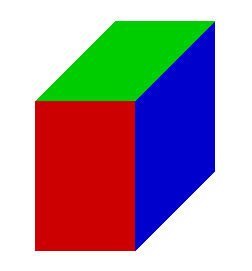# Calculate 67794

Calculate the volume of the cuboid in the given unit if you know the lengths of its edges.
A) a = 20 cm, b = 3 cm, c = 7 cm, (length)
B) a = 10 mm, b = 8 mm, c = 9 mm, (ml)
C) a = 30 cm, b = 5 cm, c = 8 cm, (l)
D) a = 300 mm, b = 4 m, c = 7 dm, (hl)

A =  4.2 dl
B =  0.72 ml
C =  1.2 l
D =  8.4 hl

### Step-by-step explanation:Did you find an error or inaccuracy? Feel free to write us. Thank you!

Tips for related online calculators
Do you know the volume and unit volume, and want to convert volume units?

#### Grade of the word problem:

We encourage you to watch this tutorial video on this math problem: